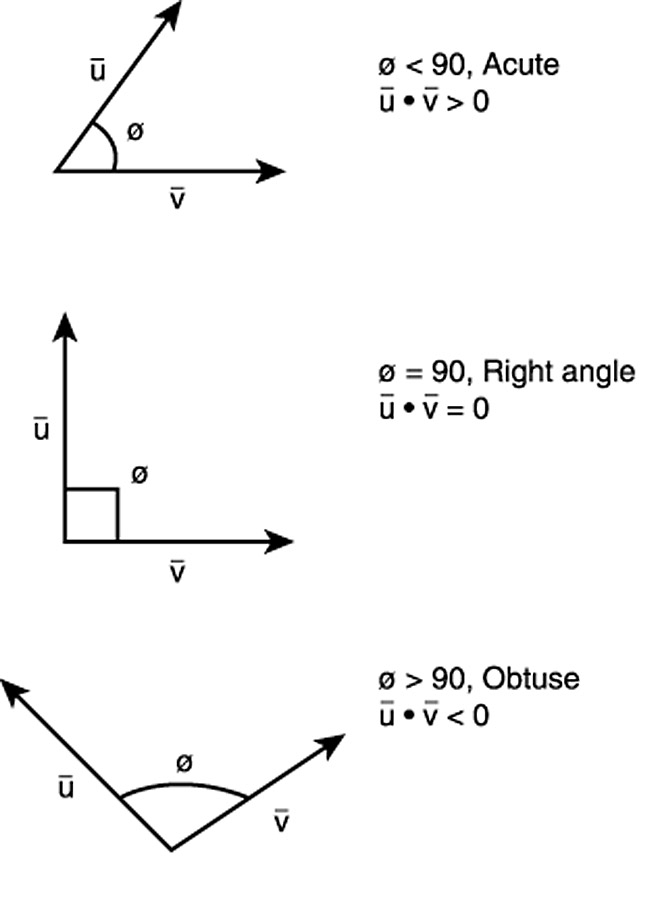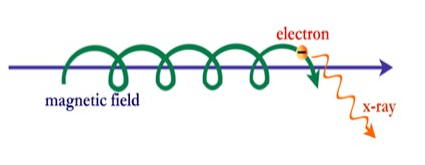## Appendix I – Vector AlgebraVector algebra is formulated to handle vectors; i.e., quantities with both magnitude and direction. Normal algebra, geometry and trigonometry are efficient at dealing with scalar quantities, that is, those with only magnitude, but are inefficient at handling vectors.  Vector algebra is an efficient way of solving 2D and 3D problems…

## Essential Guide to the EU – Chapter 4 Electromagnetism4.1  The Electromagnetic Field Equation Scientists attempt to explain physical systems in terms of mathematical models which describe and predict the behavior of the system. For example, Kepler explained the movement of the planets with his three laws. In the same way, plasma behavior is governed by the electromagnetic field…